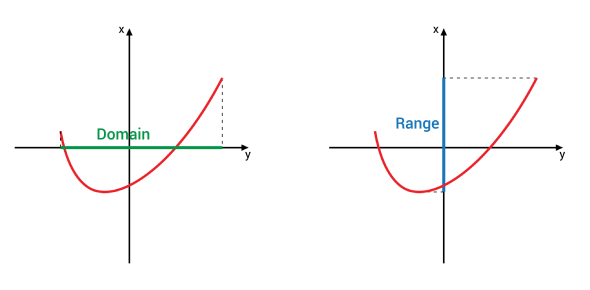# Grade 11 Domain And Range Of A Function Quiz

10 Questions | Attempts: 862SettingsHave you been practicing the domain and range of a function? Take this quiz, and see how well you perform in this quiz with the domain and range of a function. This quiz will test not only your knowledge but also your knowledge. So, you must go for this quiz and practice the math questions on the domain and range of a function. All the best for a perfect score on this quiz with all the correct answers. Do not forget to share the quiz with others math experts.

• 1.
What is the range of a linear function with a negative slope?
• A.

R = {y € R | y = –1}

• B.

R = {y € R | y < 0 }

• C.

R = {y € R }

• D.

R = {y € R | y ≠ 0}

• 2.
What are the domain and range of the function h(x) = x2 + 3?
• A.

D = {x € R}, R = {y € R }

• B.

D = {x € R | 0

• C.

D = {x € R, x => 3}, R = {y € R }

• D.

D = {x € R}, R = {y € R | y => 3 }

• 3.
A crow drops a stick from the air onto the ground.  The height of the stick above the ground after it has been release is modeled by the function h(t) = 45 – 5t2, where h(t) is the height in meters and t is time in seconds.  What is the domain of the function?
• A.

D = {t € R | 0

• B.

D = {t = 3}

• C.

D = {t € R | 0

• D.

D = {t € R | t < 3}

• 4.
A cable company charges \$116 for installation and \$36 per month.  What is the domain and range for this function?
• A.

D = {t € R | 0

• B.

D = {x € R | 0

• C.

D = {x € R }, R = {f(x) € R }

• D.

D = {t € R | 0 0}

• 5.
Bowl–o–Mat charges \$3.50 per person per game plus \$2 per person for show rental.  What is the domain of this situation?
• A.

Number of players

• B.

Total cost per game

• C.

Number of games

• D.

Cost of shoes

• 6.
Kylie is making a rectangular picture frame.  She has 84 cm of ribbon to trim the edges of the frame.  She will not use ribbon on the bottom edge of the frame.  What is the range for the function of the area of the frame?
• A.

R = {0

• B.

R = {21

• C.

R = {0

• D.

R = {42

• 7.
What is the domain and range of the quadratic function represented by the following table of values?
• A.

D = {x € R | 0

• B.

D = {x € R | –8

• C.

D = {x € R | –5

• D.

D = {x € R | –5

• 8.
Which graph shows the range R = {y € R | y => 1}
• A.

Y = (x + 1)^2 + 2

• B.

Y = (x – 2)^2 + 1

• C.

Y = 1

• D.

Y = (x – 1)^2 + 1

• 9.
A golf ball is hit out of a sand trap.  The height of the ball is modeled by the function h(t) = –5t2 + 6t – 1, where t is time in seconds.  What is the maximum height height of the gold ball?
• A.

0.6m

• B.

0.8m

• C.

1.0m

• D.

8.0m

• 10.
For the function {(0,1), (1,-3), (2,-4), (-4,1)}, write the domain and range.
• A.

D: {1, -3, -4,} R: {0, 1, 2, -4}

• B.

D:{0, 1, 2, -4} R:{1, -3, -4}

• C.

D:{0, 1, 2, 3, 4} R:{1, -3, -4}

• D.

D:{1, 2, 3, 4, 5} R:{-1, 0, 1, -3, -4}

## Related TopicsBack to top
×

Wait!
Here's an interesting quiz for you.